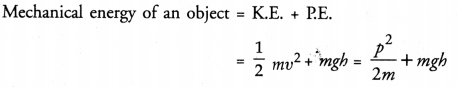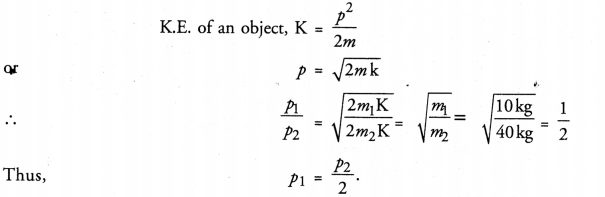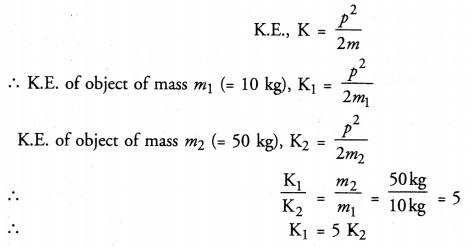## Online Education HOTS Questions for Class 9 Science Chapter 11 Work, Power and Energy

These Solutions are part of Online Education HOTS Questions for Class 9 Science. Here we have given HOTS Questions for Class 9 Science Chapter 11 Work, Power and Energy

Question 1.
A light and a heavy object have the same momentum. What is the ratio of their kinetic energy ?
(CBSE 2011, 2012)More Resources

Question 2.
Can any object have mechanical energy even if its momentum is zero ? Explain.
(NCERT Question Bank, CBSE 2011)p = 0, then mechanical energy of the object = mgh = P.E.
Thus, a stationary object at a height h above the surface of earth has mechanical energy even if its momentum is zero.

Question 3.
Can any object have momentum even if its mechanical energy is zero ? Explain.
(NCERT Question Bank ; CBSE 2011)
Mechanical energy = K.E. + P.E.
Since mechanical energy is zero, so both K.E. and P.E are zero. Kinetic energy is zero means velocity of the object is zero. Hence, momentum of the object is also zero.

Question 4.
Compare the momentum of two objects of mass 10 kg and 40 kg respectively but having same kinetic energy.